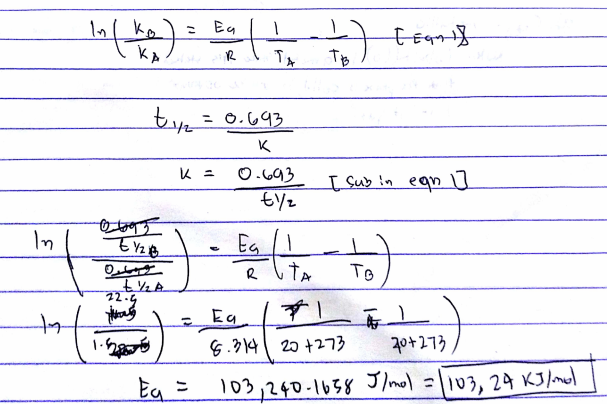# Problem: For the first-order reaction, t1/2 = 22.5 h at 20 ºC and 1.5 h at 40 ºC. N2O5 (g) → 2NO2 (g) + 1/2 O2 (g)            a) Calculate the activation energy of this reaction in kJ/mol. b) If the Arrhenius constant A = 2.05 x 10 13 s-1, determine the value of k at 29 ºC in s  -1.

###### FREE Expert Solution
98% (375 ratings)
###### FREE Expert Solution98% (375 ratings)###### Problem Details

For the first-order reaction, t1/2 = 22.5 h at 20 ºC and 1.5 h at 40 ºC.

N2O5 (g) → 2NO2 (g) + 1/2 O2 (g)

a) Calculate the activation energy of this reaction in kJ/mol.

b) If the Arrhenius constant A = 2.05 x 10 13 s-1, determine the value of k at 29 ºC in s  -1.

What scientific concept do you need to know in order to solve this problem?

Our tutors have indicated that to solve this problem you will need to apply the Arrhenius Equation concept. You can view video lessons to learn Arrhenius Equation. Or if you need more Arrhenius Equation practice, you can also practice Arrhenius Equation practice problems.

What is the difficulty of this problem?

Our tutors rated the difficulty ofFor the first-order reaction, t1/2 = 22.5 h at 20 ºC and 1.5...as medium difficulty.

How long does this problem take to solve?

Our expert Chemistry tutor, Sabrina took 13 minutes and 33 seconds to solve this problem. You can follow their steps in the video explanation above.

What professor is this problem relevant for?

Based on our data, we think this problem is relevant for Professor Polanco's class at UF.